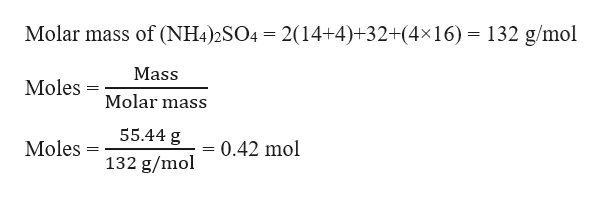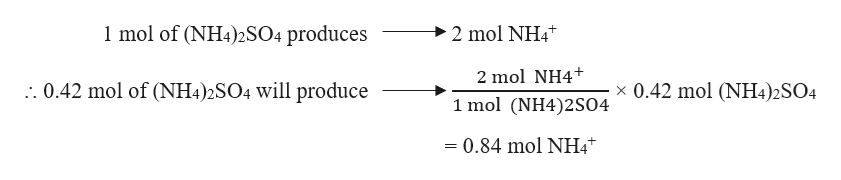# A solution of ammonium sulfate was prepared by dissolving 55.44g of (NH4)2SO4 in enough water to prepare a 2.00L solution. What is the concentration of ammonium ions in this solution?

Question
16 views

A solution of ammonium sulfate was prepared by dissolving 55.44g of (NH4)2SO4 in enough water to prepare a 2.00L solution. What is the concentration of ammonium ions in this solution?

check_circle

Step 1

Given,

Mass of (NH4)2SO4 = 55.44 g

Volume of solution = 2.00 L

The number of moles of (NH4)2SO4 can be calculated as:help_outlineImage TranscriptioncloseMolar mass of (NH4)2SO4 2(14+4)+32+(4x16) 132 g/mol Mass Moles = Molar mass 55.44 g Moles 0.42 mol 132 g/mol fullscreen
Step 2

Ammonium sulfate in water will dissociate into ammonium and sulfate ions. The balanced reaction can be written as:

Step 3

From the reaction, it i...help_outlineImage Transcriptionclose1 mol of (NH4)2SO4 produces 2 mol NH4 2 mol NH4 :. 0.42 mol of (NH4)2SO4 will produce x 0.42 mol (NH4)2SO4 1 mol (NH4)2S04 = 0.84 mol NH4 fullscreen

### Want to see the full answer?

See Solution

#### Want to see this answer and more?

Solutions are written by subject experts who are available 24/7. Questions are typically answered within 1 hour.*

See Solution
*Response times may vary by subject and question.
Tagged in

### Solutions